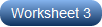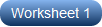# Solving Quadratic Equations by Taking Square Roots Worksheets

Unlike the standard form: ax2 + bx + c = 0, most of the quadratic equations offered in this pack of printable high school worksheets have no middle term. Such equations are known as pure quadratic equations and are of the form ax2 - c = 0. Solving these quadratic equations is made a lot easier by by taking square roots. Let's now get into the process! Here’s all you have to do. Isolate the leading term on the left-hand side of the equation and the constant term on the right-hand side, take square roots on both sides, and simplify both sides for the values of x. The equations that have the middle term can also be solved by finding square roots. Yet again, the middle term is excluded by using the appropriate algebraic identities. Get started with our free worksheet!

Push-start your practice of finding the real and complex roots of quadratic equations with this set of pdf worksheets presenting 30 pure quadratic equations. Note that the coefficient of the leading term is 1 in every equation. Hence, simply rewrite the given equation in the form of x2 = c, and proceed to solve for x.Keep one jump ahead of your peers with this printable practice set perfect for high school students! The quadratic equations here involve integers and fractions. You need to rewrite the equation to the desired form, isolate the x2 term, take square roots, and perform simplification on both sides.